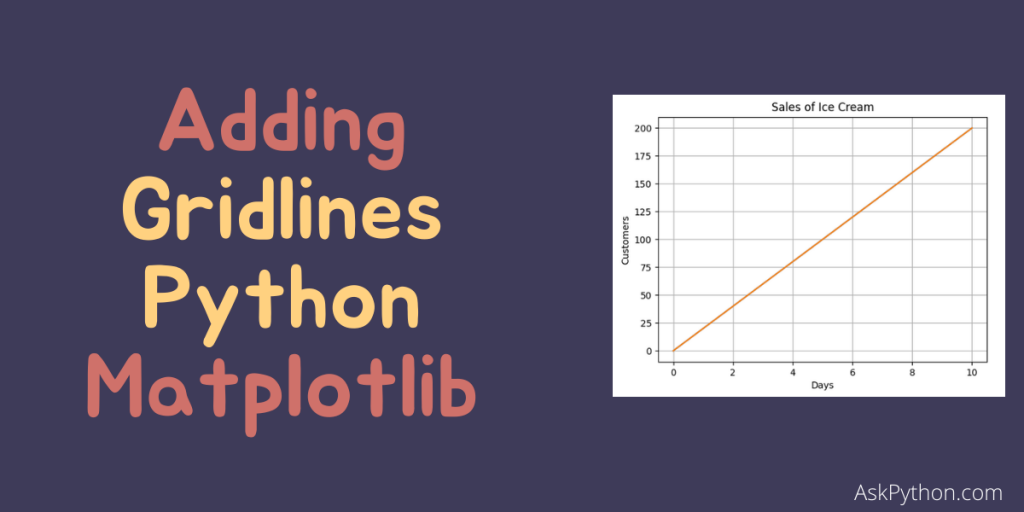# How to Add Grid Lines in Matplotlib?In this article, we’ll learn how to add gridlines in Matplotlib plots. Matplotlib is a python plotting library which provides an interactive environment for creating scientific plots and graphs. Let’s get right into the topic.

## Steps to add grid lines to Matplot lib plots

Let’s now go over the steps to add grid lines to a Matplotlib plot.

### 1. Installing the module

Matplotlib

```pip install matplotlib
```

Pyplot

The pyplot submodule contains the majority of Matplotlib’s functionality

Note: Compilers usually don’t have the ability to show graphs but in Python, we can make them compatible by adding a few lines of code:

```import sys
import matplotlib
matplotlib.use('Agg')
# Matplotlib relies on a backend to render the plots and here ‘Agg’ is the default backend

import matplotlib.pyplot as pyt
# lines of code for plotting a graph

pyt.savefig(sys.stdout.buffer)
sys.stdout.flush()
# these two lines are used to avoid excess buffering and print the data without any delay and make sure the code works
```

Example:

```import sys
import matplotlib
matplotlib.use('Agg')
# Matplotlib relies on a backend to render the plots and here ‘Agg’ is the default backend

import matplotlib.pyplot as pyt
import numpy as np

x = np.array([0, 10])
y = np.array([0, 200])

pyt.plot(x, y)

pyt.show()

pyt.savefig(sys.stdout.buffer)
sys.stdout.flush()
```

### 2. Adding Grid Lines to a plot

We can use the grid() function with Pyplot to add grid lines to a plot.

Example:

```x = np.array([0,10])
y = np.array([0,200])
pyt.title("Sales of Ice Cream")
# to represent the title on the plot

pyt.xlabel("Days")                      # to label the x-axis
pyt.ylabel("Customers")            # to label the y-axis
pyt.plot(x, y)
pyt.grid()
pyt.show()
```

### 3. Specify the Grid Lines to Display

Using the axis parameter in the grid() function, we can specify which grid lines to display. Permitted values are: ‘x’, ‘y’ or ‘both’. But the default is ‘both’ so we can avoid writing it.

Example:

• Display only x-axis grid lines:
```pyt.grid(axis = ‘y’)
```
• Display only y-axis grid lines:
```pyt.grid(axis = ‘x’)
```

### 4. Setting Line properties for the Grid

We can set the properties of the grid in various ways for color, style, etc.

We define the styling as : color= ’specify_color’, linestyle=’specify_linestyle’, linewidth= number, axis=’specify_axis(‘x’,’y’ or ‘both’)’

For example:

```pyt.grid(color = 'red', linestyle = '--', linewidth = 0.75, axis='both')
```